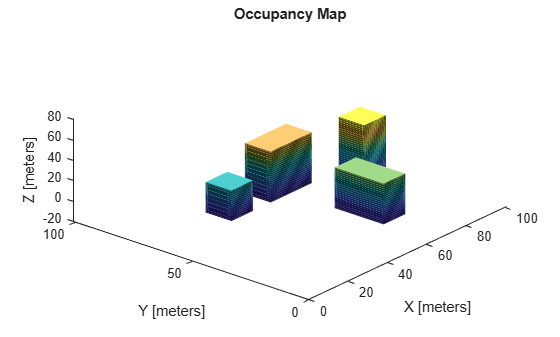# getOccupancy

Get occupancy probability of locations

## Syntax

``occval = getOccupancy(map3D,xyz)``

## Description

example

````occval = getOccupancy(map3D,xyz)` returns an array of probability occupancy values at the specified xyz locations in the `occupancyMap3D` object. Values close to 1 represent a high certainty that the cell contains an obstacle. Values close to 0 represent certainty that the cell is not occupied and obstacle-free.```

## Examples

collapse all

Import a 3-D occupancy map.

`map3D = importOccupancyMap3D("citymap.ot")`
```map3D = occupancyMap3D with properties: ProbabilitySaturation: [1.0000e-03 0.9990] Resolution: 1 OccupiedThreshold: 0.6500 FreeThreshold: 0.2000 ```

Display the map.

`show(map3D)`Check the occupancy statuses of different locations and get their occupancy values.

`iOccVal1 = checkOccupancy(map3D,[50 15 0])`
```iOccVal1 = 0 ```
`OccVal1 = getOccupancy(map3D,[50 15 0])`
```OccVal1 = 0.0019 ```
`iOccVal2 = checkOccupancy(map3D,[50 15 15])`
```iOccVal2 = 1 ```
`OccVal2 = getOccupancy(map3D,[50 15 15])`
```OccVal2 = 0.6500 ```
`iOccVal3 = checkOccupancy(map3D,[50 15 45])`
```iOccVal3 = -1 ```
`OccVal3 = getOccupancy(map3D,[50 15 45])`
```OccVal3 = 0.5000 ```

## Input Arguments

collapse all

3-D occupancy map, specified as an `occupancyMap3D` object.

World coordinates, specified as an n-by-3 matrix of `[x y z]` points, where n is the number of world coordinates.

## Output Arguments

collapse all

Probability occupancy values, returned as a column vector with the same length as `xyz`.

Values close to 0 represent certainty that the cell is not occupied and obstacle-free.

Introduced in R2019b